/

### Calibrating probability distributions used for risk measurement purposes to market-implied data: 3. Other comments

Appendix

3.1          If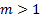then the simultaneous equations generated by the above procedure do not appear to have analytic solutions, see e.g. the Appendix. Instead they must in general be solved numerically by some iterative root search algorithm, e.g. one that finds the value of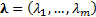that satisfies the following equation: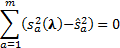where: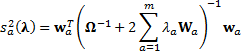If the problem involves a relatively small number of instruments/calibration implieds then the problem can be solved using relatively straightforward tools such as the Excel Solver add-in. For larger instrument universes and/or calibration sets, more sophisticated root searching algorithms may be needed.

3.2          However, it may not always be appropriate to adopt a rote formulaic approach to calibration. For example, suppose we were trying to calibrate a UK equity risk model. Easily observable implied volatilities are available from listed equity derivatives on the FTSE100 index, the FTSE250 index but only for an incomplete range of individual equities. What should we do for securities for which there are no readily available implied volatilities?

3.3          The problem of incomplete or missing data is, of course, a generic problem with calibration, and not specific to the above problem. We could of course calibrate solely to those instrument volatilities that are easily observable. However, this would typically disproportionately affect the volatility assigned to the instruments included in the calibration set, see Appendix. Suppose that in fact general levels of implied volatilities are materially higher than those in the uncalibrated prior distribution, e.g. because there is an overall perception within the market that the “world is uncertain at the moment”. Would we want calibration disproportionately to mark up volatilities of securities on which there were readily available option prices versus those on which there were not?

3.4          An alternative would perhaps be to introduce just two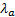’s, i.e. two calibration equations. One might calibrate the volatility of the main market index to its current implied volatility (or actually in the spirit of above the variance to its current implied variance). The other might calibrate the average volatility (variance) of individual instruments to the average of their individual implied volatilities (variances) to the extent that these exist, with the same overall volatility (variance) adjustment then also applied to instruments where there is no observable implied volatility. Or perhaps we could adopt an intermediate approach of applying averaging within individual sectors rather than across the market as a whole. Of course, such averaging approaches would be less effective at calibrating individual instrument volatilities so that they exactly matched their own implied volatilities where these exist. That is the nature of averaging! We might also want to impose further constraints on the calibration to force retention of any parsimonious factor structure imposed on the prior distribution.

3.5          Analytical weighted Monte Carlo can also be used to calibrate risk models to cater for different future time periods, as long as there is a suitable term structure of implied volatilities available. We merely need to repeat the exercise separately for each term. Again, if necessary, ‘missing’ calibration data can be handled using averaging approaches as per 3.4.

3.6          It is worth noting that calibration of risk models to market implieds may make resulting ex ante tracking errors, VaR’s and other similar risk measures more volatile (because implied volatilities are themselves volatile over even quite short periods of time). This may be important if explicit ex ante tracking error or VaR style risk limits are present in investment management agreements.  Use of risk statistics calibrated to market implieds might then create greater likelihood of breach of such mandate restrictions merely because of market movements. We could dampen the impact of this ‘volatility of volatility’ by applying some sort of credibility weighting to current implied volatilities versus volatilities extrapolated from past history but of course only at the expense of calibration quality. Alternatively, it might be appropriate to quote more than one set of risk statistics, e.g. some ‘longer term’ ones (perhaps based solely on extrapolating past history using a relatively long time window) and some ‘shorter-term’ ones more fully calibrated to market implieds.  The former might then be used more for mandate limit purposes and the latter more for day-to-day management of the portfolio.